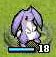# 星之一角

What have you found for these years?

## 2009-12-10

Two examples of lazy evaluation

1306. 11-30 powerset (2)

`module AvgTree wheredata Tree a = Leaf a            | Node a (Tree a) (Tree a)            deriving ShowavgTree :: (Fractional a) => Tree a -> Tree aavgTree t = result where  (result, sum, count) = avgTree' t  avg = sum / count  avgTree' (Leaf n) = (Leaf avg, n, 1)  avgTree' (Node n a b) = (Node avg left right,                           n + suma + sumb,                           1 + counta + countb) where    (left,  suma, counta) = avgTree' a    (right, sumb, countb) = avgTree' b--              Node 6.0 (Leaf 6.0) (Node 6.0 (Leaf 6.0) (Leaf 6.0))test = avgTree (Node 6   (Leaf 7)   (Node 8   (Leaf 9)   (Leaf 0)))`

#### 0 retries: